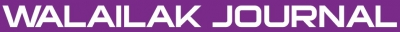### Comparative Analysis of MHD Boundary-Layer Flow of Viscoelastic Fluid in Permeable Channel with Slip Boundaries by using HAM, VIM, HPM

Morteza ABBASI, Davood Domiri GANJI, Iman RAHIMIPETROUDI, Mehran KHAKI

#### Abstract

In this present study, the problem of two-dimensional magnetohydrodynamic (MHD) boundary layer flow of steady, laminar flow of an incompressible, viscoelastic fluid in a parallel plate channel with slip at the boundaries is presented. The upper convected Maxwell model is implemented due to its accuracy in simulating highly elastic fluid flows at high Deborah numbers. Moreover, this paper deals with the solution of third order of nonlinear ordinary differential equations which are solved by using three analytical approximate methods, namely the Homotopy Analysis Method (HAM), Homotopy Perturbation Method (HPM), and Variational Iteration Method (VIM). The comparisons of these results reveal that HAM is very effective, convenient and quite accurate for non-linear ordinary differential equation. In addition, this work demonstrates that HAM is able to solve problems with mixed (Robin) as well as other boundary conditions.

doi:10.14456/WJST.2014.10

#### Keywords

Homotopy Analysis Method, Homotopy Perturbation Method, Variation Iteration Method, slip condition, magneto hydrodynamic, UCM

PDF

#### References

AH Nayfeh. Perturbation Methods. Wiley, New York, 2000.

JH He. A coupling method of homotopy technique and perturbation technique for nonlinear problems. Inter. J. Nonlinear Mech. 2000; 35, 37-43.

JH He. Homotopy perturbation method for bifurcation of nonlinear problems. Int. J. Nonlinear Sci. Numer. Simul. 2005; 6, 207-8.

JH He. Application of homotopy perturbation method to nonlinear wave equations. Chaos Soliton. Fract. 2005; 26, 695-700.

DD Ganji. The application of He’s homotopy perturbation method to nonlinear equations arising in heat transfer. Phys. Lett. A 2006; 355, 337-41.

DD Ganji and A Sadighi. Application of homotopy-perturbation and variational iteration methods to nonlinear heat transfer and porous media equations. J. Comput. Appl. Math. 2007; 207, 24-34.

JH He. Variational iteration method- some recent results and new interpretations. J. Comput. Appl. Math. 2007; 207, 3-17.

S Momani and S Abuasad. Application of He’s variational iteration method to Helmholtz equation. Chaos Soliton. Fract. 2006; 27, 1119-23.

DD Ganji, GA Afrouzi and RA Talarposhti. Application of variational iteration method and homotopy-perturbation method for nonlinear heat diffusion and heat transfer equations. Phys. Lett. A 2007; 368, 450-7.

JH He. Variational iteration method- a kind of nonlinear analytical technique: Some examples. Int. J. Nonlinear Mech. 1999; 34, 699-708.

JH He. Approximate analytical solution for seepage with fractional derivatives in porous media. Comput. Meth. Appl. Mech. Eng. 1998; 167, 57-68.

SJ Liao. Homotopy Analysis Method in Nonlinear Differential Equation. Berlin & Beijing, Springer & Higher Education Press, 2012.

SJ Liao. Beyond Perturbation: Introduction to the Homotopy Analysis Method. Chapman & Hall/CRC Press, Boca Raton, 2003.

M Turkyilmazoglu. Purely analytic solutions of magnetohydrodynamic swirling boundary layer flow over a porous rotating disk. Comput. Fluid. 2010; 39, 793-9.

M Turkyilmazoglu. Analytic approximate solutions of rotating disk boundary layer flow subject to a uniform suction or injection. Int. J. Mech. Sci. 2010; 52, 1735-44.

M Esmaeilpour and DD Ganji. Solution of the Jeffery-Hamel flow problem by optimal homotopy asymptotic method. Comput. Math. Appl. 2010; 59, 3405-11.

SJ Lio. An analytic solution of unsteady boundary-layer flows caused by an impulsively stretching plate. Commun. Nonlinear Sci. Numer. Simulat. 2006; 11, 326-39.

SJ Lio. A new branch of solutions of boundary layer flows over an incompressible stretching plate. Int. J. Heat Mass Trans. 2005; 48, 2529-39.

SJ Liao. A short review on the homotopy analysis method in fluid mechanics. J. Hydrodyn. 2010; 22, 882-4.

G Domairry and M Fazeli. Homotopy analysis method to determine the fin efficiency of convective straight fins with temperature dependent thermal conductivity. Commun. Nonlinear Sci. Numer. Simulat. 2009; 14, 489-99.

MM Rashidi, G Domairry and S Dinarvand. Approximate solutions for the Burger and regularized long wave equations by means of the Homotopy analysis method. Commun. Nonlinear Sci. Numer. Simulat. 2009; 14, 708-17.

Z Ziabakhsh, G Domairry and M Esmaeilpour. Solution of the laminar viscous flow in a semi-porous channel in the presence of a uniform magnetic field by using the Homotopy analysis method. Commun. Nonlinear Sci. Numer. Simulat. 2009; 14, 1284-94.

MM Rashidi and S Dinarvand. Purely analytic approximate solutions for steady three-dimensional problem of condensation film on inclined rotating disk by Homotopy analysis method. Nonlinear Anal. R. World Appl. 2009; 10, 2346-56.

Z Ziabakhsh and G Domairry. Analytic solution of natural convection flow of a non-Newtonian fluid between two vertical flat plates using Homotopy analysis method. Commun. Nonlinear Sci. Numer. Simulat. 2009; 14, 1868-80.

M Turkyilmazoglu. A note on the homotopy analysis method. Appl. Math. Lett. 2010; 23, 1226-30.

M Turkyilmazoglu. Numerical and analytical solutions for the flow and heat transfer near the equator of an MHD boundary layer over a porous rotating sphere. Int. J. Therm. Sci. 2011; 50, 831-42.

CS Liu. The essence of the generalized Newton binomial theorem. Commun. Nolinear Sci. Numer. Simulat. 2010; 15, 2766-8.

CS Liu. The essence of the homotopy analysis method. Appl. Math. Comput. 2010; 216, 1299-303.

CS Liu and Y Liu. Comparison of the general series method and the homotopy analysis method. Mod. Phys. Lett. B 2010; 24, 1699-706.

CS Liu. The essence of the generalized Taylor theorem as the foundation of the homotopy analysis method. Commun. Nonlinear Sci. Numer. Simulat. 2011; 16, 1254-62.

OA Bég and OD Makinde. Viscoelasticflow and species transfer in a Darcian high-permeability channel. J. Petrol. Sci. Eng. 2010; 76, 93-9.

T Hayat and Z Abbas. Channel flow of a Maxwell fluid with chemical reaction. J. Appl. Math. Phys. 2008; 59, 124-44.

A Heck. Introduction to Maple. 2nd ed. Springer, New York, USA, 1996.

### Refbacks

• There are currently no refbacks.Online ISSN: 2228-835X

http://wjst.wu.ac.th

Last updated: 20 June 2019